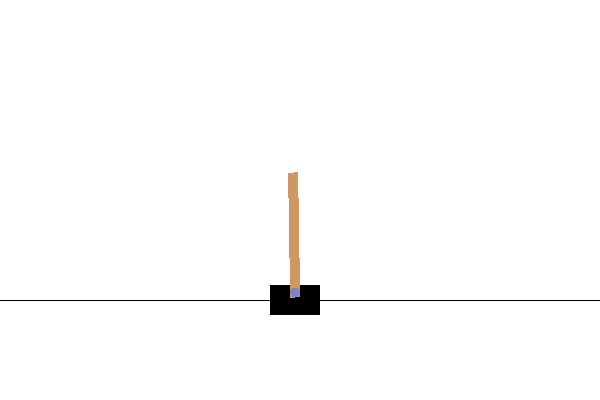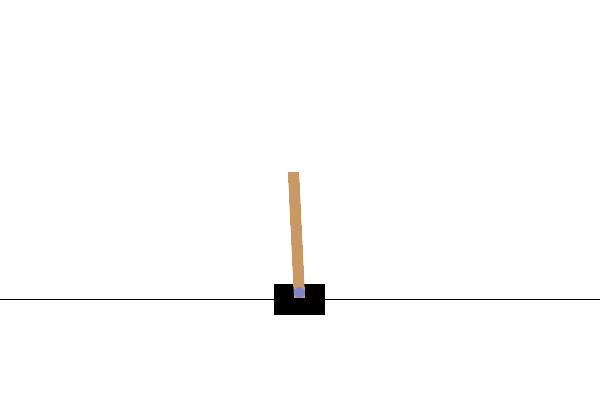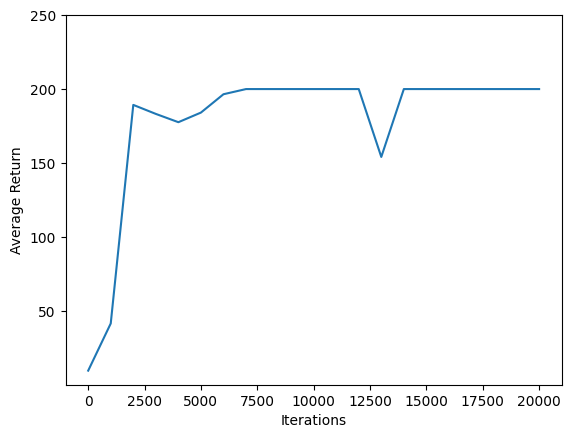# Train a Deep Q Network with TF-Agents

## Introduction

This example shows how to train a DQN (Deep Q Networks) agent on the Cartpole environment using the TF-Agents library.It will walk you through all the components in a Reinforcement Learning (RL) pipeline for training, evaluation and data collection.

To run this code live, click the 'Run in Google Colab' link above.

## Setup

If you haven't installed the following dependencies, run:

````sudo apt-get install -y xvfb ffmpeg`
`pip install -q 'gym==0.10.11'`
`pip install -q 'imageio==2.4.0'`
`pip install -q PILLOW`
`pip install -q 'pyglet==1.3.2'`
`pip install -q pyvirtualdisplay`
`pip install -q tf-agents`
```
```

0 upgraded, 0 newly installed, 0 to remove and 108 not upgraded.

```
``````from __future__ import absolute_import, division, print_function

import base64
import imageio
import IPython
import matplotlib
import matplotlib.pyplot as plt
import numpy as np
import PIL.Image
import pyvirtualdisplay

import tensorflow as tf

from tf_agents.agents.dqn import dqn_agent
from tf_agents.drivers import dynamic_step_driver
from tf_agents.environments import suite_gym
from tf_agents.environments import tf_py_environment
from tf_agents.eval import metric_utils
from tf_agents.metrics import tf_metrics
from tf_agents.networks import q_network
from tf_agents.policies import random_tf_policy
from tf_agents.replay_buffers import tf_uniform_replay_buffer
from tf_agents.trajectories import trajectory
from tf_agents.utils import common
``````
``````tf.compat.v1.enable_v2_behavior()

# Set up a virtual display for rendering OpenAI gym environments.
display = pyvirtualdisplay.Display(visible=0, size=(1400, 900)).start()
``````
``````tf.version.VERSION
``````
```'2.2.0'
```

## Hyperparameters

``````num_iterations = 20000 # @param {type:"integer"}

initial_collect_steps = 1000  # @param {type:"integer"}
collect_steps_per_iteration = 1  # @param {type:"integer"}
replay_buffer_max_length = 100000  # @param {type:"integer"}

batch_size = 64  # @param {type:"integer"}
learning_rate = 1e-3  # @param {type:"number"}
log_interval = 200  # @param {type:"integer"}

num_eval_episodes = 10  # @param {type:"integer"}
eval_interval = 1000  # @param {type:"integer"}
``````

## Environment

In Reinforcement Learning (RL), an environment represents the task or problem to be solved. Standard environments can be created in TF-Agents using `tf_agents.environments` suites. TF-Agents has suites for loading environments from sources such as the OpenAI Gym, Atari, and DM Control.

Load the CartPole environment from the OpenAI Gym suite.

``````env_name = 'CartPole-v0'
``````

You can render this environment to see how it looks. A free-swinging pole is attached to a cart. The goal is to move the cart right or left in order to keep the pole pointing up.

``````
env.reset()
PIL.Image.fromarray(env.render())
``````The `environment.step` method takes an `action` in the environment and returns a `TimeStep` tuple containing the next observation of the environment and the reward for the action.

The `time_step_spec()` method returns the specification for the `TimeStep` tuple. Its `observation` attribute shows the shape of observations, the data types, and the ranges of allowed values. The `reward` attribute shows the same details for the reward.

``````print('Observation Spec:')
print(env.time_step_spec().observation)
``````
```Observation Spec:
BoundedArraySpec(shape=(4,), dtype=dtype('float32'), name='observation', minimum=[-4.8000002e+00 -3.4028235e+38 -4.1887903e-01 -3.4028235e+38], maximum=[4.8000002e+00 3.4028235e+38 4.1887903e-01 3.4028235e+38])

```
``````print('Reward Spec:')
print(env.time_step_spec().reward)
``````
```Reward Spec:
ArraySpec(shape=(), dtype=dtype('float32'), name='reward')

```

The `action_spec()` method returns the shape, data types, and allowed values of valid actions.

``````print('Action Spec:')
print(env.action_spec())
``````
```Action Spec:
BoundedArraySpec(shape=(), dtype=dtype('int64'), name='action', minimum=0, maximum=1)

```

In the Cartpole environment:

• `observation` is an array of 4 floats:
• the position and velocity of the cart
• the angular position and velocity of the pole
• `reward` is a scalar float value
• `action` is a scalar integer with only two possible values:
• `0` — "move left"
• `1` — "move right"
``````time_step = env.reset()
print('Time step:')
print(time_step)

action = np.array(1, dtype=np.int32)

next_time_step = env.step(action)
print('Next time step:')
print(next_time_step)
``````
```Time step:
TimeStep(step_type=array(0, dtype=int32), reward=array(0., dtype=float32), discount=array(1., dtype=float32), observation=array([ 0.03362545,  0.00367401, -0.00529881,  0.02672447], dtype=float32))
Next time step:
TimeStep(step_type=array(1, dtype=int32), reward=array(1., dtype=float32), discount=array(1., dtype=float32), observation=array([ 0.03369893,  0.19887155, -0.00476432, -0.26762557], dtype=float32))

```

Usually two environments are instantiated: one for training and one for evaluation.

``````train_py_env = suite_gym.load(env_name)
``````

The Cartpole environment, like most environments, is written in pure Python. This is converted to TensorFlow using the `TFPyEnvironment` wrapper.

The original environment's API uses Numpy arrays. The `TFPyEnvironment` converts these to `Tensors` to make it compatible with Tensorflow agents and policies.

``````train_env = tf_py_environment.TFPyEnvironment(train_py_env)
eval_env = tf_py_environment.TFPyEnvironment(eval_py_env)
``````

## Agent

The algorithm used to solve an RL problem is represented by an `Agent`. TF-Agents provides standard implementations of a variety of `Agents`, including:

The DQN agent can be used in any environment which has a discrete action space.

At the heart of a DQN Agent is a `QNetwork`, a neural network model that can learn to predict `QValues` (expected returns) for all actions, given an observation from the environment.

Use `tf_agents.networks.q_network` to create a `QNetwork`, passing in the `observation_spec`, `action_spec`, and a tuple describing the number and size of the model's hidden layers.

``````fc_layer_params = (100,)

q_net = q_network.QNetwork(
train_env.observation_spec(),
train_env.action_spec(),
fc_layer_params=fc_layer_params)
``````

Now use `tf_agents.agents.dqn.dqn_agent` to instantiate a `DqnAgent`. In addition to the `time_step_spec`, `action_spec` and the QNetwork, the agent constructor also requires an optimizer (in this case, `AdamOptimizer`), a loss function, and an integer step counter.

``````optimizer = tf.compat.v1.train.AdamOptimizer(learning_rate=learning_rate)

train_step_counter = tf.Variable(0)

agent = dqn_agent.DqnAgent(
train_env.time_step_spec(),
train_env.action_spec(),
q_network=q_net,
optimizer=optimizer,
td_errors_loss_fn=common.element_wise_squared_loss,
train_step_counter=train_step_counter)

agent.initialize()
``````

## Policies

A policy defines the way an agent acts in an environment. Typically, the goal of reinforcement learning is to train the underlying model until the policy produces the desired outcome.

In this tutorial:

• The desired outcome is keeping the pole balanced upright over the cart.
• The policy returns an action (left or right) for each `time_step` observation.

Agents contain two policies:

• `agent.policy` — The main policy that is used for evaluation and deployment.
• `agent.collect_policy` — A second policy that is used for data collection.
``````eval_policy = agent.policy
collect_policy = agent.collect_policy
``````

Policies can be created independently of agents. For example, use `tf_agents.policies.random_tf_policy` to create a policy which will randomly select an action for each `time_step`.

``````random_policy = random_tf_policy.RandomTFPolicy(train_env.time_step_spec(),
train_env.action_spec())
``````

To get an action from a policy, call the `policy.action(time_step)` method. The `time_step` contains the observation from the environment. This method returns a `PolicyStep`, which is a named tuple with three components:

• `action` — the action to be taken (in this case, `0` or `1`)
• `state` — used for stateful (that is, RNN-based) policies
• `info` — auxiliary data, such as log probabilities of actions
``````example_environment = tf_py_environment.TFPyEnvironment(
``````
``````time_step = example_environment.reset()
``````
``````random_policy.action(time_step)
``````
```PolicyStep(action=<tf.Tensor: shape=(1,), dtype=int64, numpy=array()>, state=(), info=())
```

## Metrics and Evaluation

The most common metric used to evaluate a policy is the average return. The return is the sum of rewards obtained while running a policy in an environment for an episode. Several episodes are run, creating an average return.

The following function computes the average return of a policy, given the policy, environment, and a number of episodes.

``````
def compute_avg_return(environment, policy, num_episodes=10):

total_return = 0.0
for _ in range(num_episodes):

time_step = environment.reset()
episode_return = 0.0

while not time_step.is_last():
action_step = policy.action(time_step)
time_step = environment.step(action_step.action)
episode_return += time_step.reward
total_return += episode_return

avg_return = total_return / num_episodes
return avg_return.numpy()

# See also the metrics module for standard implementations of different metrics.
# https://github.com/tensorflow/agents/tree/master/tf_agents/metrics
``````

Running this computation on the `random_policy` shows a baseline performance in the environment.

``````compute_avg_return(eval_env, random_policy, num_eval_episodes)
``````
```26.9
```

## Replay Buffer

The replay buffer keeps track of data collected from the environment. This tutorial uses `tf_agents.replay_buffers.tf_uniform_replay_buffer.TFUniformReplayBuffer`, as it is the most common.

The constructor requires the specs for the data it will be collecting. This is available from the agent using the `collect_data_spec` method. The batch size and maximum buffer length are also required.

``````replay_buffer = tf_uniform_replay_buffer.TFUniformReplayBuffer(
data_spec=agent.collect_data_spec,
batch_size=train_env.batch_size,
max_length=replay_buffer_max_length)
``````

For most agents, `collect_data_spec` is a named tuple called `Trajectory`, containing the specs for observations, actions, rewards, and other items.

``````agent.collect_data_spec
``````
```Trajectory(step_type=TensorSpec(shape=(), dtype=tf.int32, name='step_type'), observation=BoundedTensorSpec(shape=(4,), dtype=tf.float32, name='observation', minimum=array([-4.8000002e+00, -3.4028235e+38, -4.1887903e-01, -3.4028235e+38],
dtype=float32), maximum=array([4.8000002e+00, 3.4028235e+38, 4.1887903e-01, 3.4028235e+38],
dtype=float32)), action=BoundedTensorSpec(shape=(), dtype=tf.int64, name='action', minimum=array(0), maximum=array(1)), policy_info=(), next_step_type=TensorSpec(shape=(), dtype=tf.int32, name='step_type'), reward=TensorSpec(shape=(), dtype=tf.float32, name='reward'), discount=BoundedTensorSpec(shape=(), dtype=tf.float32, name='discount', minimum=array(0., dtype=float32), maximum=array(1., dtype=float32)))
```
``````agent.collect_data_spec._fields
``````
```('step_type',
'observation',
'action',
'policy_info',
'next_step_type',
'reward',
'discount')
```

## Data Collection

Now execute the random policy in the environment for a few steps, recording the data in the replay buffer.

``````
def collect_step(environment, policy, buffer):
time_step = environment.current_time_step()
action_step = policy.action(time_step)
next_time_step = environment.step(action_step.action)
traj = trajectory.from_transition(time_step, action_step, next_time_step)

# Add trajectory to the replay buffer

def collect_data(env, policy, buffer, steps):
for _ in range(steps):
collect_step(env, policy, buffer)

collect_data(train_env, random_policy, replay_buffer, steps=100)

# This loop is so common in RL, that we provide standard implementations.
# For more details see the drivers module.
# https://www.tensorflow.org/agents/api_docs/python/tf_agents/drivers
``````

The replay buffer is now a collection of Trajectories.

``````# For the curious:
# Uncomment to peel one of these off and inspect it.
# iter(replay_buffer.as_dataset()).next()
``````

The agent needs access to the replay buffer. This is provided by creating an iterable `tf.data.Dataset` pipeline which will feed data to the agent.

Each row of the replay buffer only stores a single observation step. But since the DQN Agent needs both the current and next observation to compute the loss, the dataset pipeline will sample two adjacent rows for each item in the batch (`num_steps=2`).

This dataset is also optimized by running parallel calls and prefetching data.

``````# Dataset generates trajectories with shape [Bx2x...]
dataset = replay_buffer.as_dataset(
num_parallel_calls=3,
sample_batch_size=batch_size,
num_steps=2).prefetch(3)

dataset
``````
```<PrefetchDataset shapes: (Trajectory(step_type=(64, 2), observation=(64, 2, 4), action=(64, 2), policy_info=(), next_step_type=(64, 2), reward=(64, 2), discount=(64, 2)), BufferInfo(ids=(64, 2), probabilities=(64,))), types: (Trajectory(step_type=tf.int32, observation=tf.float32, action=tf.int64, policy_info=(), next_step_type=tf.int32, reward=tf.float32, discount=tf.float32), BufferInfo(ids=tf.int64, probabilities=tf.float32))>
```
``````iterator = iter(dataset)

print(iterator)

``````
```<tensorflow.python.data.ops.iterator_ops.OwnedIterator object at 0x7f3bcc1f4898>

```
``````# For the curious:
# Uncomment to see what the dataset iterator is feeding to the agent.
# Compare this representation of replay data
# to the collection of individual trajectories shown earlier.

# iterator.next()
``````

## Training the agent

Two things must happen during the training loop:

• collect data from the environment
• use that data to train the agent's neural network(s)

This example also periodicially evaluates the policy and prints the current score.

The following will take ~5 minutes to run.

``````
try:
%%time
except:
pass

# (Optional) Optimize by wrapping some of the code in a graph using TF function.
agent.train = common.function(agent.train)

# Reset the train step
agent.train_step_counter.assign(0)

# Evaluate the agent's policy once before training.
avg_return = compute_avg_return(eval_env, agent.policy, num_eval_episodes)
returns = [avg_return]

for _ in range(num_iterations):

# Collect a few steps using collect_policy and save to the replay buffer.
for _ in range(collect_steps_per_iteration):
collect_step(train_env, agent.collect_policy, replay_buffer)

# Sample a batch of data from the buffer and update the agent's network.
experience, unused_info = next(iterator)
train_loss = agent.train(experience).loss

step = agent.train_step_counter.numpy()

if step % log_interval == 0:
print('step = {0}: loss = {1}'.format(step, train_loss))

if step % eval_interval == 0:
avg_return = compute_avg_return(eval_env, agent.policy, num_eval_episodes)
print('step = {0}: Average Return = {1}'.format(step, avg_return))
returns.append(avg_return)
``````
```step = 200: loss = 7.271472930908203
step = 400: loss = 16.107458114624023
step = 600: loss = 118.40227508544922
step = 800: loss = 309.5046691894531
step = 1000: loss = 289.1570129394531
step = 1000: Average Return = 162.60000610351562
step = 1200: loss = 823.7694091796875
step = 1400: loss = 2027.1971435546875
step = 1600: loss = 946.24658203125
step = 1800: loss = 393.8977355957031
step = 2000: loss = 2000.873046875
step = 2000: Average Return = 52.599998474121094
step = 2200: loss = 175.0901641845703
step = 2400: loss = 273.25341796875
step = 2600: loss = 2591.3798828125
step = 2800: loss = 152.83265686035156
step = 3000: loss = 1936.0728759765625
step = 3000: Average Return = 26.399999618530273
step = 3200: loss = 209.44723510742188
step = 3400: loss = 1566.943115234375
step = 3600: loss = 630.6491088867188
step = 3800: loss = 767.8441772460938
step = 4000: loss = 508.98345947265625
step = 4000: Average Return = 42.599998474121094
step = 4200: loss = 752.710693359375
step = 4400: loss = 363.8679504394531
step = 4600: loss = 603.786865234375
step = 4800: loss = 28.982707977294922
step = 5000: loss = 23.120849609375
step = 5000: Average Return = 65.0
step = 5200: loss = 66.53056335449219
step = 5400: loss = 168.55474853515625
step = 5600: loss = 87.38054656982422
step = 5800: loss = 93.24928283691406
step = 6000: loss = 191.4639892578125
step = 6000: Average Return = 49.900001525878906
step = 6200: loss = 105.91390991210938
step = 6400: loss = 47.351165771484375
step = 6600: loss = 88.13796997070312
step = 6800: loss = 71.17057800292969
step = 7000: loss = 525.8966064453125
step = 7000: Average Return = 18.700000762939453
step = 7200: loss = 110.9851303100586
step = 7400: loss = 87.06258392333984
step = 7600: loss = 102.72260284423828
step = 7800: loss = 54.91393280029297
step = 8000: loss = 100.33734130859375
step = 8000: Average Return = 16.100000381469727
step = 8200: loss = 36.66551971435547
step = 8400: loss = 212.24395751953125
step = 8600: loss = 133.2703857421875
step = 8800: loss = 89.03622436523438
step = 9000: loss = 531.134521484375
step = 9000: Average Return = 33.400001525878906
step = 9200: loss = 3865.49365234375
step = 9400: loss = 95.14530944824219
step = 9600: loss = 3612.396484375
step = 9800: loss = 65.28089904785156
step = 10000: loss = 133.4741668701172
step = 10000: Average Return = 22.299999237060547
step = 10200: loss = 109.11979675292969
step = 10400: loss = 89.59709930419922
step = 10600: loss = 82.05484008789062
step = 10800: loss = 208.7845001220703
step = 11000: loss = 46.07347106933594
step = 11000: Average Return = 16.0
step = 11200: loss = 42.30530548095703
step = 11400: loss = 150.30064392089844
step = 11600: loss = 174.80587768554688
step = 11800: loss = 154.945556640625
step = 12000: loss = 8173.28369140625
step = 12000: Average Return = 67.0
step = 12200: loss = 105.27813720703125
step = 12400: loss = 187.780517578125
step = 12600: loss = 413.2526550292969
step = 12800: loss = 7414.9013671875
step = 13000: loss = 7637.66845703125
step = 13000: Average Return = 24.200000762939453
step = 13200: loss = 761.740234375
step = 13400: loss = 718.4118041992188
step = 13600: loss = 114.23948669433594
step = 13800: loss = 856.1566162109375
step = 14000: loss = 377.7228698730469
step = 14000: Average Return = 47.29999923706055
step = 14200: loss = 425.98785400390625
step = 14400: loss = 157.39088439941406
step = 14600: loss = 210.12271118164062
step = 14800: loss = 348.72637939453125
step = 15000: loss = 450.41943359375
step = 15000: Average Return = 19.200000762939453
step = 15200: loss = 91.37750244140625
step = 15400: loss = 96.49124908447266
step = 15600: loss = 96.59413146972656
step = 15800: loss = 106.01812744140625
step = 16000: loss = 140.29470825195312
step = 16000: Average Return = 20.299999237060547
step = 16200: loss = 397.5931091308594
step = 16400: loss = 208.6700897216797
step = 16600: loss = 89.70021057128906
step = 16800: loss = 103.64916229248047
step = 17000: loss = 184.55992126464844
step = 17000: Average Return = 23.5
step = 17200: loss = 82.64599609375
step = 17400: loss = 343.134033203125
step = 17600: loss = 160.8577880859375
step = 17800: loss = 19507.083984375
step = 18000: loss = 1829.780029296875
step = 18000: Average Return = 32.20000076293945
step = 18200: loss = 803.7889404296875
step = 18400: loss = 838.5554809570312
step = 18600: loss = 456.93017578125
step = 18800: loss = 912.6376953125
step = 19000: loss = 161.77871704101562
step = 19000: Average Return = 29.200000762939453
step = 19200: loss = 117.10322570800781
step = 19400: loss = 318.7609558105469
step = 19600: loss = 300.47637939453125
step = 19800: loss = 177.61358642578125
step = 20000: loss = 90.32322692871094
step = 20000: Average Return = 25.799999237060547

```

## Visualization

### Plots

Use `matplotlib.pyplot` to chart how the policy improved during training.

One iteration of `Cartpole-v0` consists of 200 time steps. The environment gives a reward of `+1` for each step the pole stays up, so the maximum return for one episode is 200. The charts shows the return increasing towards that maximum each time it is evaluated during training. (It may be a little unstable and not increase monotonically each time.)

``````

iterations = range(0, num_iterations + 1, eval_interval)
plt.plot(iterations, returns)
plt.ylabel('Average Return')
plt.xlabel('Iterations')
plt.ylim(top=250)
``````
```(6.675000095367431, 250.0)
```### Videos

Charts are nice. But more exciting is seeing an agent actually performing a task in an environment.

First, create a function to embed videos in the notebook.

``````def embed_mp4(filename):
"""Embeds an mp4 file in the notebook."""
b64 = base64.b64encode(video)
tag = '''
<video width="640" height="480" controls>
<source src="data:video/mp4;base64,{0}" type="video/mp4">
Your browser does not support the video tag.
</video>'''.format(b64.decode())

return IPython.display.HTML(tag)
``````

Now iterate through a few episodes of the Cartpole game with the agent. The underlying Python environment (the one "inside" the TensorFlow environment wrapper) provides a `render()` method, which outputs an image of the environment state. These can be collected into a video.

``````def create_policy_eval_video(policy, filename, num_episodes=5, fps=30):
filename = filename + ".mp4"
with imageio.get_writer(filename, fps=fps) as video:
for _ in range(num_episodes):
time_step = eval_env.reset()
video.append_data(eval_py_env.render())
while not time_step.is_last():
action_step = policy.action(time_step)
time_step = eval_env.step(action_step.action)
video.append_data(eval_py_env.render())
return embed_mp4(filename)

create_policy_eval_video(agent.policy, "trained-agent")
``````
```WARNING:root:IMAGEIO FFMPEG_WRITER WARNING: input image is not divisible by macro_block_size=16, resizing from (400, 600) to (400, 608) to ensure video compatibility with most codecs and players. To prevent resizing, make your input image divisible by the macro_block_size or set the macro_block_size to None (risking incompatibility). You may also see a FFMPEG warning concerning speedloss due to data not being aligned.

```

For fun, compare the trained agent (above) to an agent moving randomly. (It does not do as well.)

``````create_policy_eval_video(random_policy, "random-agent")
``````
```WARNING:root:IMAGEIO FFMPEG_WRITER WARNING: input image is not divisible by macro_block_size=16, resizing from (400, 600) to (400, 608) to ensure video compatibility with most codecs and players. To prevent resizing, make your input image divisible by the macro_block_size or set the macro_block_size to None (risking incompatibility). You may also see a FFMPEG warning concerning speedloss due to data not being aligned.

```